Sie sind hier: ICP » R. Hilfer » Publikationen

# 2 Definition of Models

[2.3.1] Consider first the integral equation of motion for the CTRW-model [9, 10]. [2.3.2] The probability density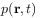obeys the integral equation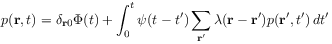(3)

where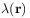denotes the probability for a displacement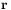in each single step, and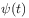is the waiting time distribution giving the probability density for the time interval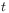between two consecutive steps. [2.3.3] The transition probabilities obey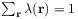, and the function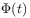is the survival probability at the initial position which is related to the waiting time distribution through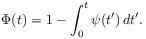(4)

[page 3, §0]   Fourier-Laplace transformation leads to the solution in Fourier-Laplace space given as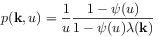(5)

where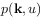is the Fourier-Laplace transform ofand similarly for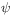and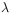.

[3.1.1] Two lattice models with different waiting time density will be considered. [3.1.2] In the first model the waiting time density is chosen as the one found in [1, 2]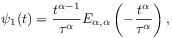(6)

where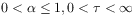is the characteristic time, and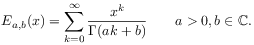(7)

is the generalized Mittag-Leffler function . [3.1.3] In the second model the waiting time density is chosen as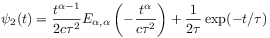(8)

where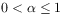,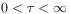and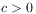is a suitable dimensional constant.

[3.2.1] The waiting time density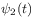differs only little from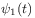as shown graphically in Figure 1.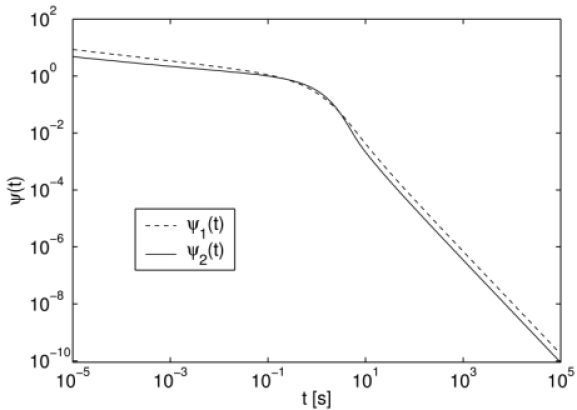Figure 1: Waiting time densitiesfor model 1 andfor model 2 with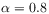,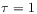s and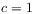s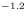.

[3.2.2] Note that both models have a long time tail of the form given in eq. (2), and the average waiting time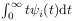diverges.

[3.3.1] For both models the spatial transition probabilities are chosen as those for nearest-neighbour transitions (Polya walk) on a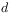-dimensional hypercubic lattice given as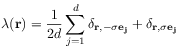(9)

where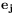is the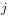-th unit basis vector generating the lattice,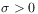is the lattice constant and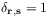for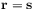and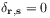for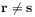.